# Texas Go Math Grade 3 Unit 1 Answer Key Number and Operations: Place Value, Fractions, Addition, and Subtraction

Refer to our Texas Go Math Grade 3 Answer Key Pdf to score good marks in the exams. Test yourself by practicing the problems from Texas Go Math Grade 3 Unit 1 Answer Key Number and Operations: Place Value, Fractions, Addition, and Subtraction.

## Texas Go Math Grade 3 Unit 1 Answer Key Number and Operations: Place Value, Fractions, Addition, and Subtraction

Show What You Know

Check your understanding of important skills.

Regroup. Write the missing numbers.

Question 1.
35 tens = ___ hundreds 5 tens

35 tens = 3 hundreds 5 tens

Question 2.
5 hundreds 3 tens = ___ tens

5 hundreds 3 tens = 53 tens

Question 3.
9 tens 10 ones = ___ hundred

9 tens 10 ones = 1 hundred

Question 4.
10 hundreds = ___ thousands

10 hundreds = 1 thousand

Equal or Unequal Parts

Question 5.
Find the shape that is divided into 2 equal parts. Color $$\frac{1}{2}$$.Question 6.
Find the shape that is divided into 4 equal parts. Color $$\frac{1}{4}$$.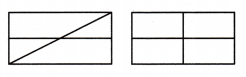Addition and Subtraction Facts to 20

Question 7.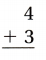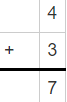Question 8.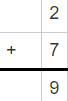Question 9.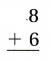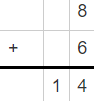Question 10.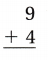Question 11.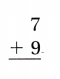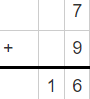Question 12.Question 13.Question 14.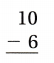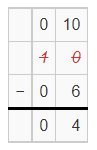Question 15.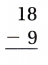Question 16.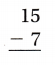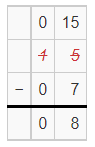Vocabulary Builder

Visualize It
Write the review words with a ✓ on the Word Line, from least to greatest place value.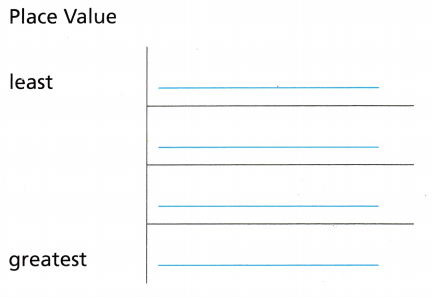Understand Vocabulary

Complete the sentences by using preview words.

Question 1.
The ___ of a number is a way to write numbers by using words.

The Numeration of a number is a way to write numbers by using words

Question 2.
The part of a fraction above the line, which tells how many parts are being counted. ____

The part of a fraction above the line, which tells how many parts are being counted is Numerator.

Question 3.
___ are numbers that are easy to compute mentally.

Compatible numbers are numbers that are easy to compute mentally.

Question 4.
___ are two fractions that name the same amount.

Equivalent fractions are two fractions that name the same amount.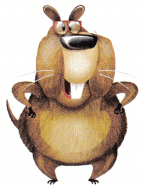Vocabulary

Mario is doing his math homework. He is getting ready to start his first addition problem. “I can solve this problem in several ways,” he says. Now it’s your turn. Find at least one way to estimate the answer and three ways to solve the problem. Show your work.

Question 1.
Estimate by rounding to the nearest tens place. Then add.Question 2.
Add by using a number line.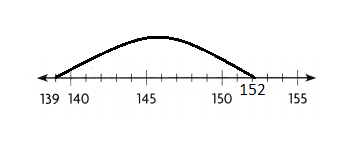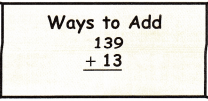Question 3.
Add by using the break apart strategy.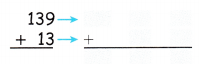Question 4.Writing What if you didn’t have paper, a pencil, or a calculator? Explain how you would use mental math to solve this problem.

Reading Look for this book in the library. Sea Sums, by Joy Hulme

Object of the Game Make two-digit addends so that their sum has a large number in the ones place.

Materials

• number cube labeled 1-6
• number cube labeled 4-9

How to Play

1. Player 1 tosses both number cubes and writes a two-digit number using the tossed numbers. Then the player tosses both cubes again and writes another number.
2. Player 1 adds the two numbers. Player 2 checks the addition. The ones digit of the sum is the number of points that Player 1 earns.
3. Players take turns repeating Steps 1 and 2. Each player keeps a record of his or her total number of points. The first player to reach a total of 50 points wins.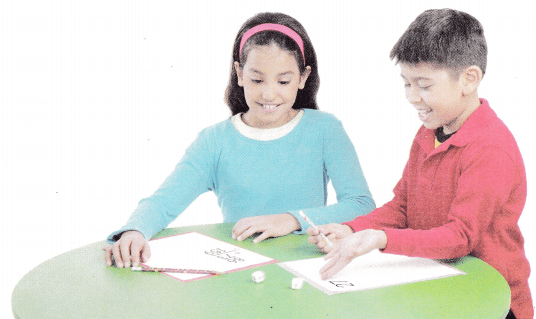Scroll to Top